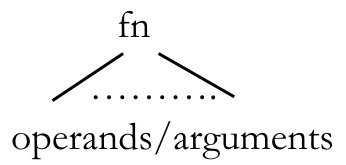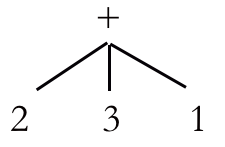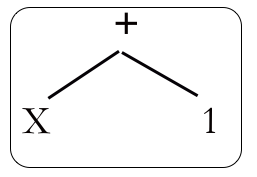# Evaluating Expressions

We’ve seen how Functional Programs can have arbitrarily long lists of operands passed to most operators and we’ve also looked at how we can manipulate lists. This section will look at how we these together to see how to evaluate these expressions.

Let’s take this expression: (- 10 3 2 1)

The algorithm for evaluating is:


Get the car and cdr

Subtract the car of the cdr from the car

Repeat while there is something in the tail



This might look a little confusing at first, but remember how important it is to be able to design code in which we have very simple functions that are executed many times. Here’s what would happen when we run this algorithm:


Get the car and cdr [That is, 10 and (3 2 1)]

Subtract the car of the cdr from the car [That is, subtract 3 from 10 to give 7]

Set the head equal to the answer [This gives us (- 7 2 1)]

Repeat while there is something in the tail [Go back to the start and repeat!]



What happens is that the list will get progressively smaller until there is nothing left except our answer. Here is each step laid out:


Expression      car      cdr     car(cdr)

(-10 3 2 1)       10    (3 2 1)      3

(- 7 2 1)        7        (2 1)      2

(- 5 1)        5           (1)      1



So we do the same thing multiple times, and only ever have to subtract two items. What’s very nice about this is we can use the same algorithm no matter what the operator is; the only difference is the operation we carry out in Step #5 above. For example, if we have (/ 20 5 4 2), then the only difference is that each time we need to do the calculation we carry out a division.

Expression      car      cdr     car(cdr)

(/ 20 5 4 2)       20    (5 4 2)      5

(/ 4 4 2)         4      (4 2)      4

(/ 1 2)        1         (2)      2



And our final answer is …

$$\frac{1}{2}$$

yes, often in Functional Programming we prefer to use fractions, as they give better precision than decimals.

What does this mean for ASTs? It means that they are smaller, because now we can have fewer operators. Our general form is now:General form of an AST

We can have multiple operands attached to a single operator, like here:Example AST with three operands

We’re still missing something, though, and that’s the ability to use variables. Remember this guy?

$$\frac{-b \pm \sqrt{b^2-4ac\;\;}}{2a}$$

What makes it useful is that although it always does the same thing, the specific way it behaves differs depending on the suer input. Let’s say we had this simple AST:A simple AST with one variable.

How do we tell it what value we want that AST to have? The answer, my friends, lies in the magic of Lambda Calculus!!